## Another question about AC analysis with Multiple Sources

 Hello there, I started trying out Circuit Lab and I think it's awesome! I started trying to do a problem using AC analysis that it's not that straightforward. I browsed around the forums and found out that CL is not setup to do this for now but... I saw some work arounds: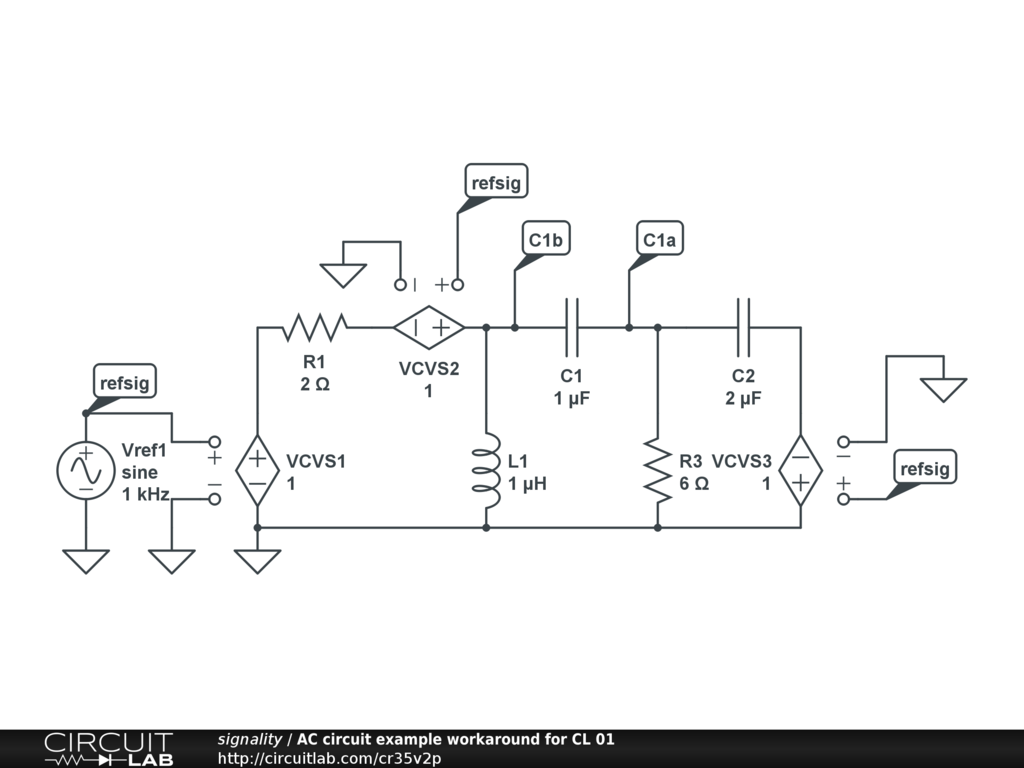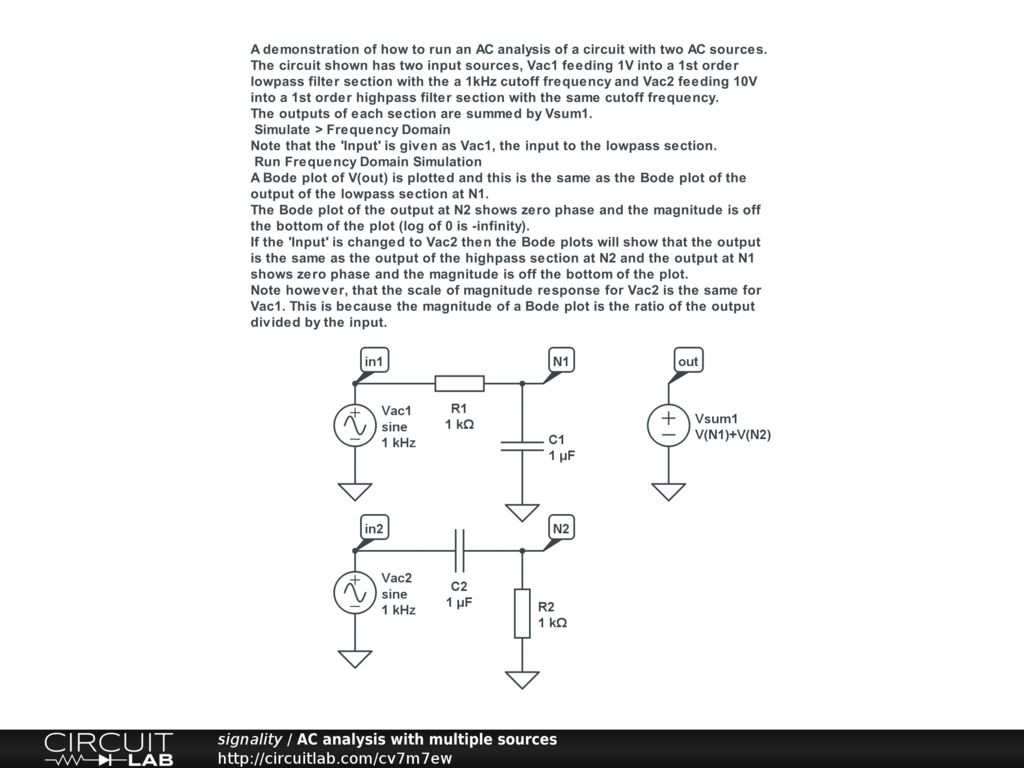I couldn't find anything that could do a problem like the one below that has both a current and voltage source at the same frequency...Could I use CL find the magnitude and phase of "i" at the frequency of 2/(2*PI)--> 318m Hz? Please let me know if there is a work around. Thanks! by Robox November 15, 2012 If you read the notes in:carefully and make sure you understand exactly what it is telling you then you can solve your problem by applying that workaround technique to your circuit. Because current into the nA end of a 2 pin component is of the opposite sign to that into the opposite nB end, you must take special care to note which end of the cap (call it C1 in your simulation) that the CCCS is monitoring and which you plot. If you run a frequency domain simulation then the stop frequency must be > the start frequency but if you run it from 0.318Hz to, say, 0.318001Hz and plot MAG(I(C1.nA)) and PHDEG(I(C1.nA)) then you will get a magnitude and phase dot on the plot showing you the required values. You can examine these with the cursor. You'll find the current controlled current Source (CCCS) under Controlled Sources in the left hand Editor panel. And here's how to use it: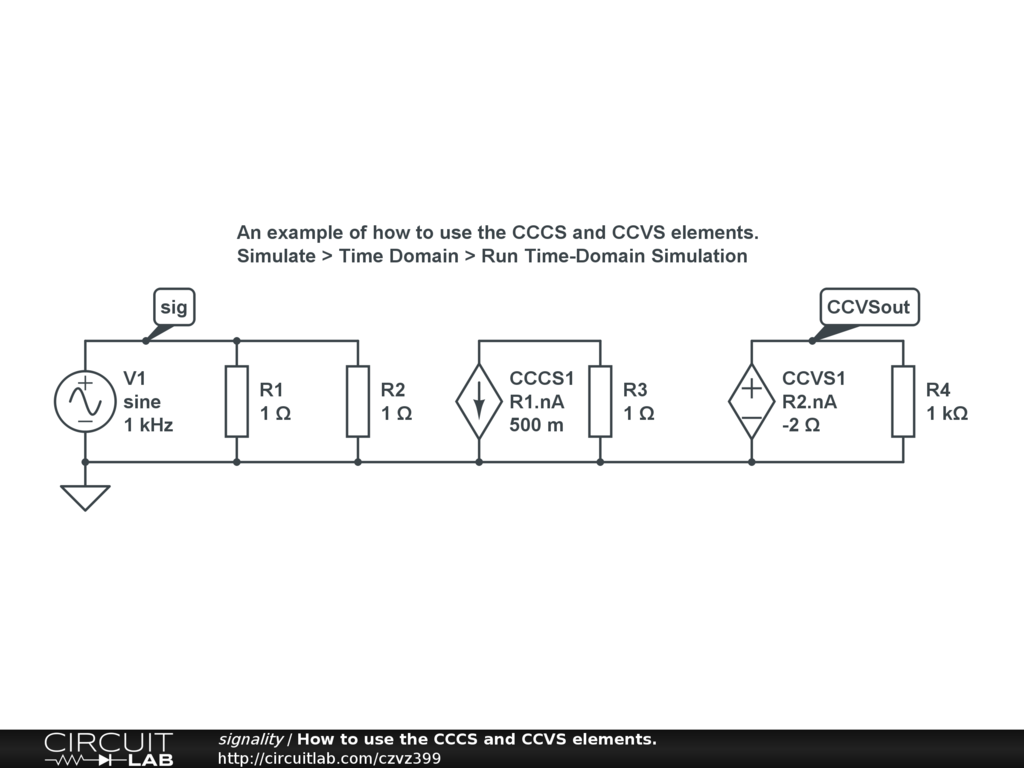by signality November 16, 2012 Thanks @signality, So how would I represent two sources that are out of phase by 90 degrees (20sin2t & 5cos2t in the above examples) using just one main source and manipulating CCCS and CCVS elements since they only scale in magnitude and not in phase? I'm assuming I would have to create two sources for sine and cosine waves? You do this in your second problem above but you have to do two separate analysis for both sources. I'm trying to find away where the correct answer just shows up by using just one simulation. Am I assuming something wrong here? Thanks by Robox November 16, 2012 Hi @signality, I thought of a work around by adding a differentiator op amp to create the phase shift.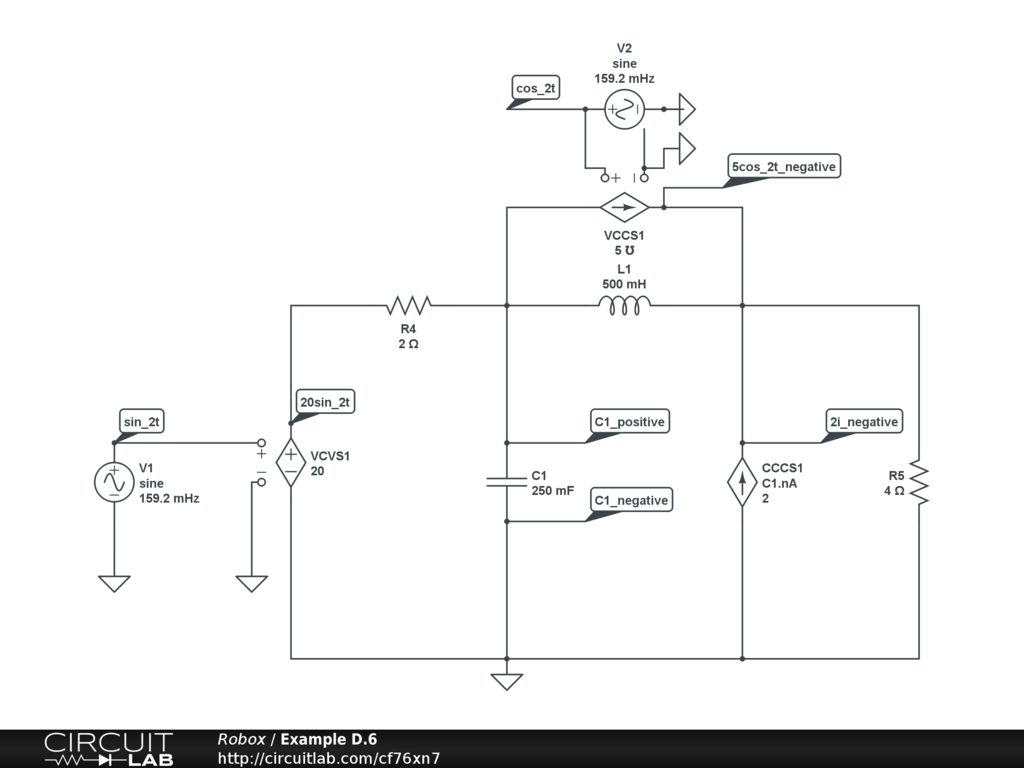I'm still not getting the correct solution of the current at the capacitor f=318mHz I should get a magnitude of 7.906A with a Phase Angle of 43.49 degrees. When I check all CCCS and CCVS elements they are showing the correct wave form so I don't really understand what I did wrong? by Robox November 16, 2012 Thanks @signality, I tried to do what you said but still no luck.by Robox November 17, 2012 Well actually I somewhat get the answer when I add up the currents from both analysis of the sources. Capacitor current from analysis of V1 = 7.071A Capacitor current from analysis of V2 = 857.5mA Circuit Lab Total current = 7.9285A Pspice current = 7.906A Assuming this is the right way of getting the current. I would I get the phase? I just can't add them up. by Robox November 17, 2012 OK PLEASE DISREGARD THE LAST TWO POSTS I GET IT NOW! Ok so basically we're essentially using superposition theorem and then adding up the currents. Since we are dealing with phasors we have to break them up into their rectangular form then add them up. So everything in the problem worked out except one thing that I can't get around? The phasor for V1 should = 7.071<45o = 5+j5 What I get is off by 90o --> 7.071<135o = -5+j5The phasor for V2 = 0.8575<30.96o = 0.7353 + j0.4412 which is what I get with PSpice In order for the answer to work V1 and V2 have to be in the same quadrant in order to add up to 5.735+j5.441 --> 7.906<43.49o Am I not assuming something correct with V1? Should have I shifted something by 90o? by Robox November 17, 2012 Think it's because you have set the phase of V2 to -90 instead of +90. Here's the solution I prepared earlier for comparison: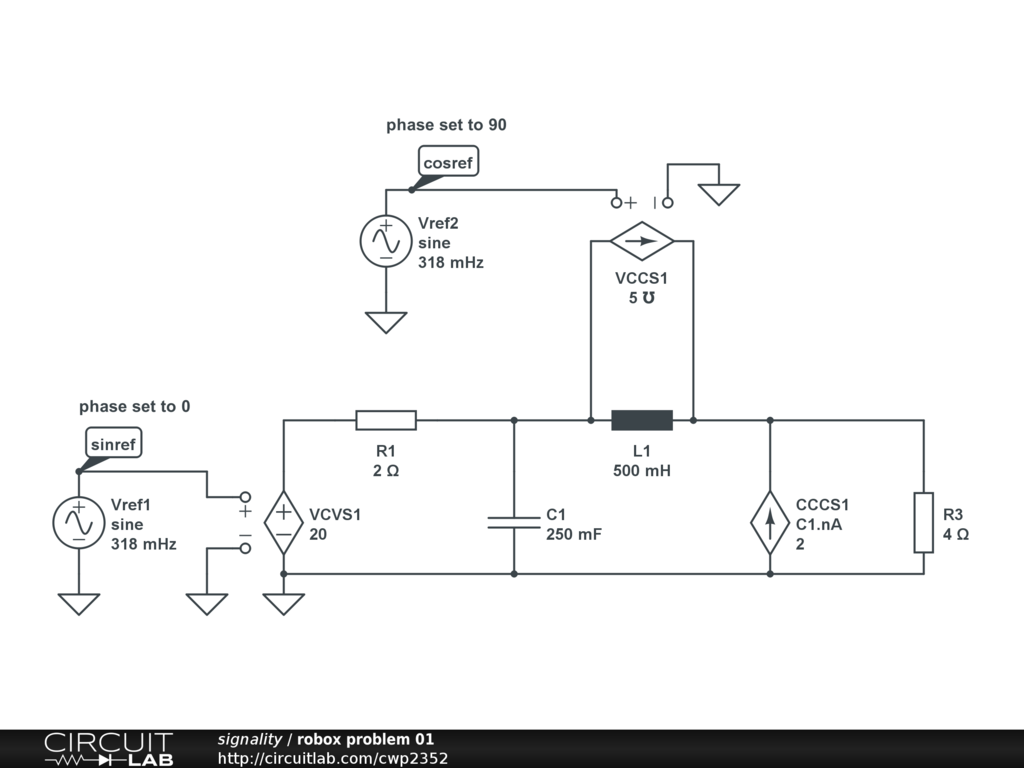by signality November 18, 2012 No problem... I have OCD lol, Yeah I'm not sure how you report bugs on CL as I'm new to the site. Is the forums the only way to do it? I wrote them an email asking them a question but they never got back to me....oh well. Btw this bug happens doesn't happen all the time: Here's a problem that it worked for perfectly.Here you have to frequencies you need to manually input. V1 = 796mHz V2 = 1.592 Hz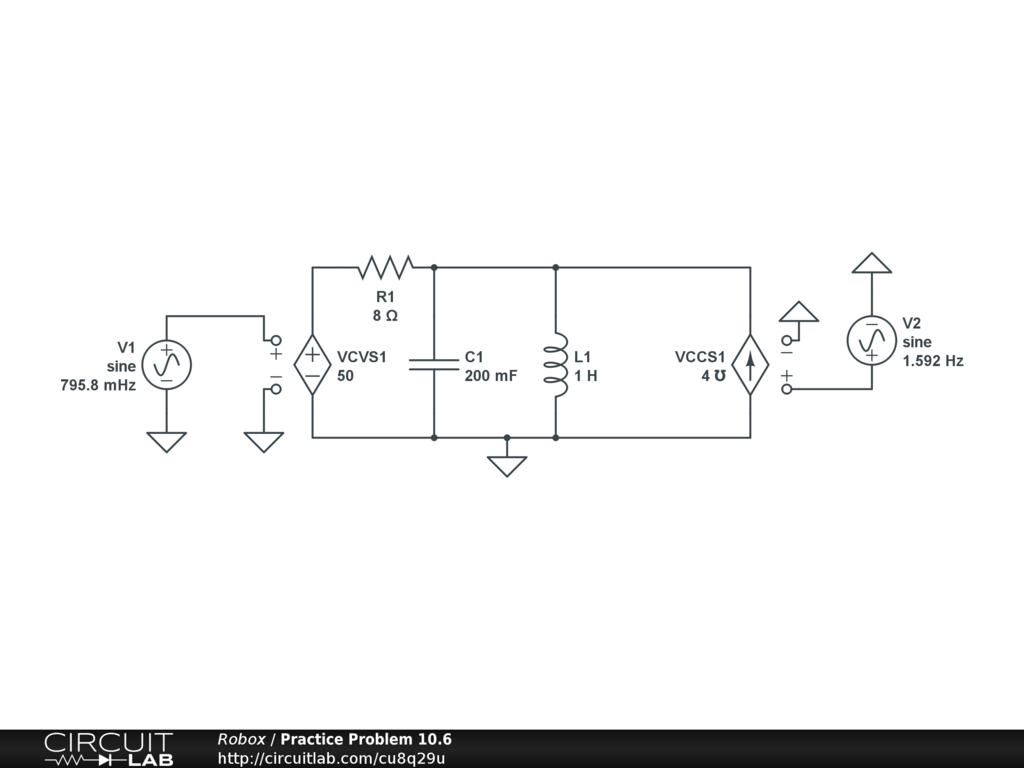The problem works out perfectly in this case. Maybe it's was just a coincidence where the math just worked out in this case. Yeah it's gotta be a bug for sure. by Robox November 19, 2012 I've posted a bug report: https://www.circuitlab.com/forums/support/topic/z6pmkkcd/bug-report-phase-in-frequency-domain-simulation-is-wrong/ Here's how: https://www.circuitlab.com/forums/support/topic/8s9n9hav/how-to-use-the-circuitlab-support-forum/ A little late now but welcome to CL! by signality November 20, 2012 Glad to join! I love this comment. "If CL is going to continue to be used in the classroom, especially at university degree course level, then it must be able to handle this sort of problem correctly." LIKE A BOSS by Robox November 20, 2012 Hi @Robox and @signality, Just wanted to let you know that we've just added a little more flexibility to the AC analysis input specifier which should solve these issues. In the "Frequency Domain" tab, under the "Input" specifier where you'd normally type "V1" or "Vref1", you can now optionally specify one or more phasors, for example, "V1 20 -90 I1 5 0", which specifies V1 with magnitude 20 and -90 degrees phase and I1 with magnitude 5 and phase 0. The attempts earlier in this discussion about using superposition and running the simulation twice and scaling/rotating/summing the complex results were definiely on the right track! In fact, that's basically what this new input phasor specification does: modifies the complex stimulus vector that's the input to CircuitLab's frequency-domain solver. (Note that the voltage/current function generator elements and their amplitude/phase parameters still are not the sources for AC analysis.) Here's my version of the original question: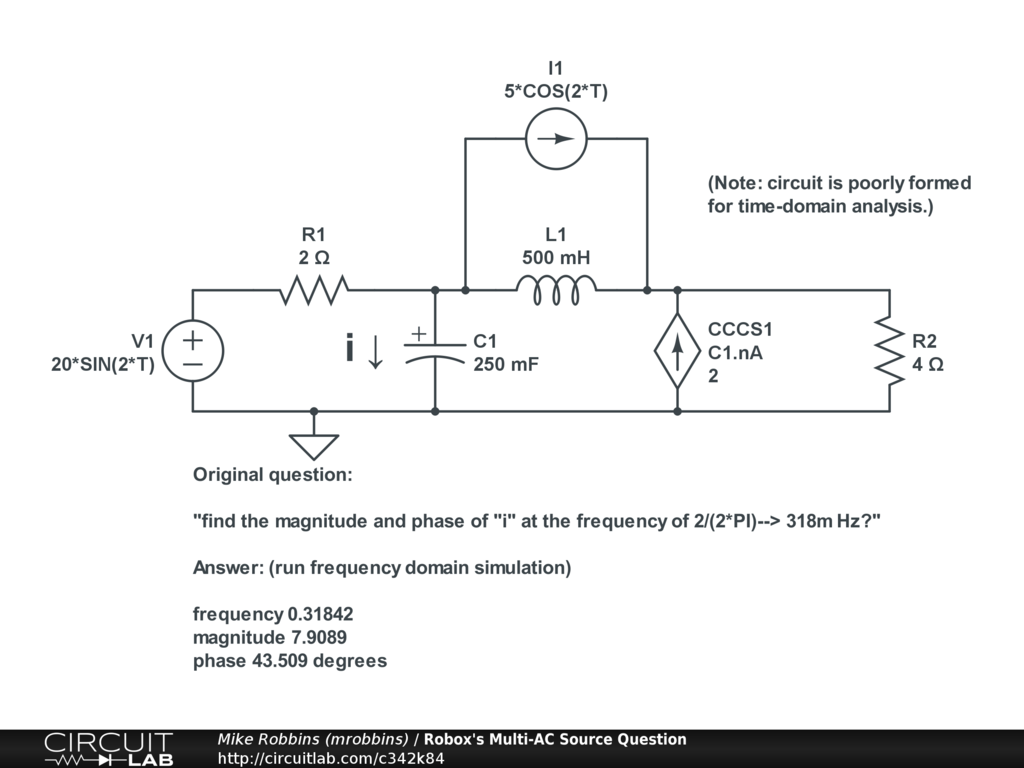Run the frequency-domain sim and you'll see the correct magnitude and phase (7.91 amps at 43.5 degrees phase), matching the textbook and other simulators, with the input specified as "V1 20 -90 I1 5 0". Also wanted to point out that this circuit doesn't simulate properly in the time domain, but that's because the circuit as shown is actually unstable due to the dependent current source! We can talk about "loop gain" and "return ratio", but basically, the loop from the dependent current source to the the terminal/quantity that it's dependent on is an unstable positive feedback loop. Here's a demo of using CircuitLab to quantify the loop gain as a function of frequency: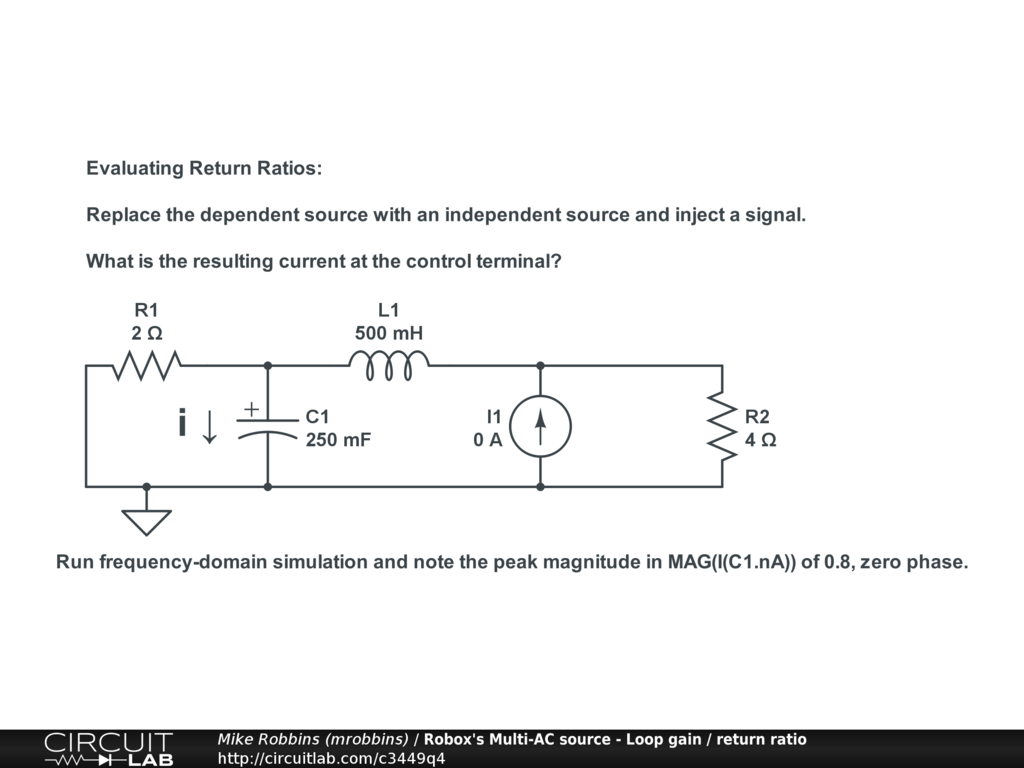Hope that helps! Happy holidays all. by mrobbins December 20, 2012